# How to Count Cells that Contain negative Numbers in Excel

This post will guide you how to count the number of cells that contain negative numbers within a range of cells using a formula in Excel 2013/2016.You can count the number of negative numbers in your data using easy functions in Excel. And the below will show you two ways in which you can count negative numbers in Excel.

## Count Number of Cells that Contain negative Numbers

Assuming that you have a data list in the range of cells B1:B6, and you want count the number of negative numbers, and write the result in cell D1.

If you want to count the number of cells that contain negative numbers in range B1:B6, and you can use a formula based on the COUNTIF function.  Like this:

=COUNTIF(B1:B6,”<0″)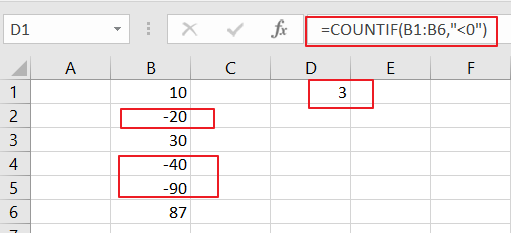LET’S SEE THAT HOW THIS FORMULA WORKS:

The COUNTIF function will be used to count the number of cells in a range that match a specified criteria. So you need to put your range of cells that containing the data you want to the function to count the number. And it goes through each cell in the range B1:B6 and counts only those cells that match the condition. Then return final result in Cell D1.

So if you want to count the number of negative numbers through COUNTIF function, the condition should be “<0”.when a number is less that 0, it should be considered as negative number.

## Count Number of Cells that Contain negative Numbers using VBA Macro

You can also use an Excel VBA Macro to count the number of negative numbers in a selected range, just do the following steps:

Step1# open your excel workbook and then click on “Visual Basic” command under DEVELOPER Tab, or just press “ALT+F11” shortcut.Step2#  then the “Visual Basic Editor” window will appear.

Step3# click “Insert” ->”Module” to create a new module.Step4# paste the below VBA code into the code window. Then clicking “Save” button.

```Sub CountNegativeNumbers()
Dim mysheet As Worksheet
Dim myrange As Range
Dim finalResult As Range
Set mysheet = Application.ActiveSheet
Set myrange = Application.Selection
Set finalResult = Application.InputBox(Title:="select a range that you want to count", Prompt:="Select one cell that you want the result to appear", Type:=8)
finalResult.Value = Application.WorksheetFunction.CountIf(myrange, "<0")
End Sub

```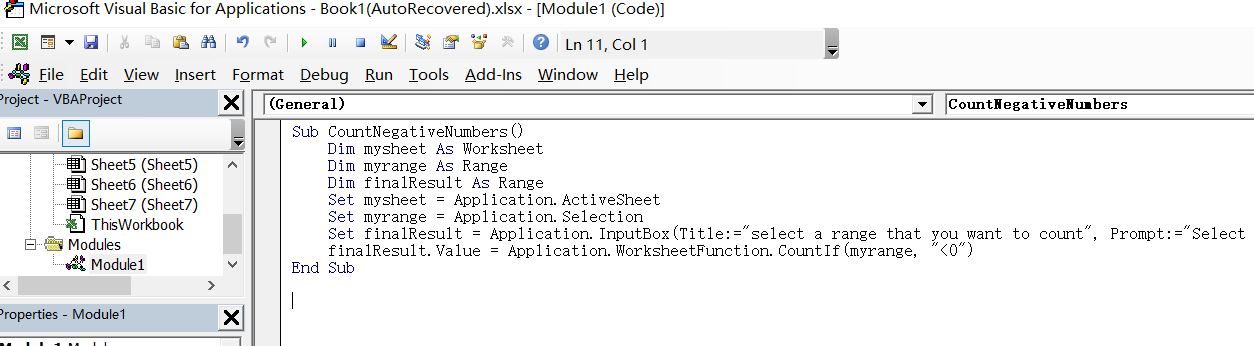Step5# select one range of cells that you want to count. such as: B1:B6.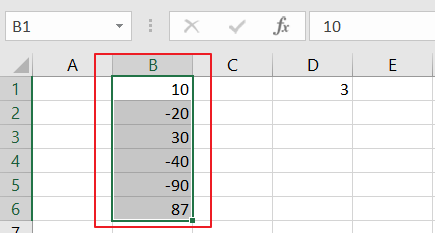Step6# back to the current worksheet, click on `Macros` button under `Code` group. then click `Run` button.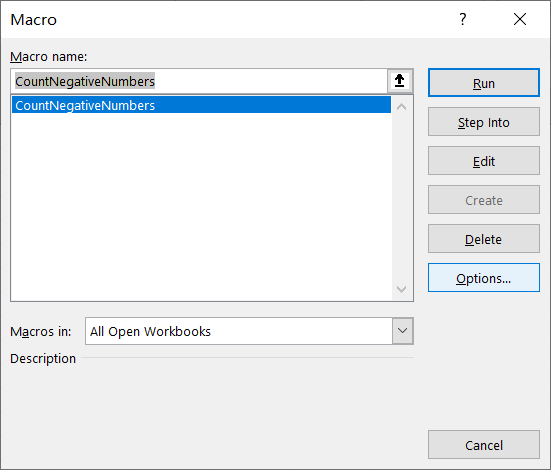Step7# select one cell that you want the final result to appear.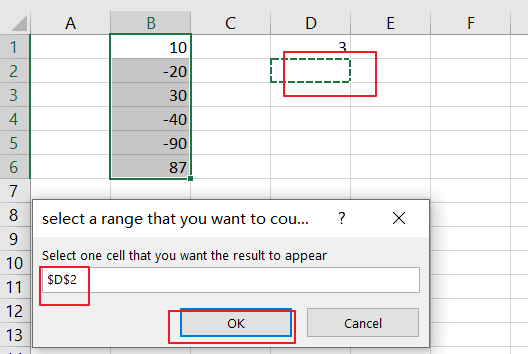Step8# let’s see the result: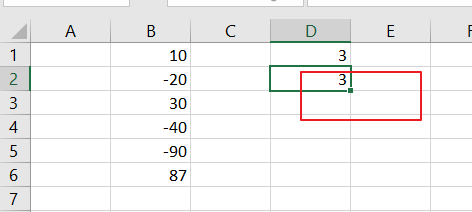### Related Functions

• Excel COUNTIF function
The Excel COUNTIF function will count the number of cells in a range that meet a given criteria. This function can be used to count the different kinds of cells with number, date, text values, blank, non-blanks, or containing specific characters.etc.= COUNTIF (range, criteria)…